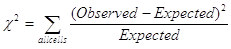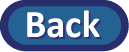## Summary

### Technology Skills learned in this lab

• Generating a segmented bar graph in the Multiple Proportions applet
• Performing a chi-square test in the Multiple Proportions applet

### Statistical Skills learned in this lab

• (Review) Describing the association between two categorical variables
• Stating the hypotheses for a chi-square test (in terms of several population proportions or association between variables)
• Calculating a chi-square test statistic as a measure of the association between two categorical variables. Note: This statistic generalizes to more columns and rows in the two-way table much more easily than summing pairwise differences. The general formula iswhere "Expected" are the expected cell counts under the null hypothesis (e.g., applying the overall proportions of successes to each sample size and then for failures too).
• Keep in mind, the Chi-square distribution is valid as long as the cell counts are large enough.
• Interpreting a p-value in terms of shulffing the response variable values.